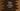# Python program to check if a series is Arithmetic progression or not## Python program to check if a series is Arithmetic progression or not:

In this post, we will learn how to check if a series is Arithmetic progression series or not. The program will take one series of numbers and print one message that this is an Arithmetic progression or not.

For example, 1, 3, 5, 7, 9, 11 is an Arithmetic progression, but 2, 4, 7, 8 is not.

## Algorithm to check for AP:

For an Arithmetic progression or AP, each numbers are separated by a constant value. This value is called common difference.

So, we can check if the common difference or if the difference between two numbers are equal or not for all values.

We can follow these steps to check if a series is an Arithmetic progression or not:

• Find the difference between the first and the second number and store it in a variable.
• Start from the third number and for each number,

• Check if the difference between this number and its previous number is equal to the difference we checked in the first step.
• If the difference is not equal, this is not an AP..
• If the difference is equal, move to the next number.
• If all differences are equal, this is an Arithmetic progression.

### Python program:

Below is the complete python program:

``````def check_arithmetic_progression(arr):
diff = arr - arr
n = len(arr)

for i in range(2, n):
if arr[i] - arr[i - 1] != diff:
return False

return True

print(check_arithmetic_progression([1, 3, 5, 7, 9, 11]))
print(check_arithmetic_progression([1, 3, 5, 7, 9, 11, 12]))
print(check_arithmetic_progression([1, 3, 5, 7, 9, 11, 13, 15]))
print(check_arithmetic_progression([5, 10, 11, 12]))``````

Here,

• checkarithmeticprogression method is used to check if an array is an Arithmetic progression or not.
• It calculates the difference between the second and the first element and store that value in diff. This is the common difference that we want for all places in the series.
• The for loop starts from the index 2, or from the third element of the array to the end of the array.
• For each number, it calculates the difference or common difference by subtracting the previous number from the current number.

• It calculates if the difference is equal to the calculated diff or not. If not, it returns False.
• Once the for loop ends, it returns True, because all numbers have equal common difference.

### Output:

If you run this program, it will print the below output:

``````True
False
True
False``````